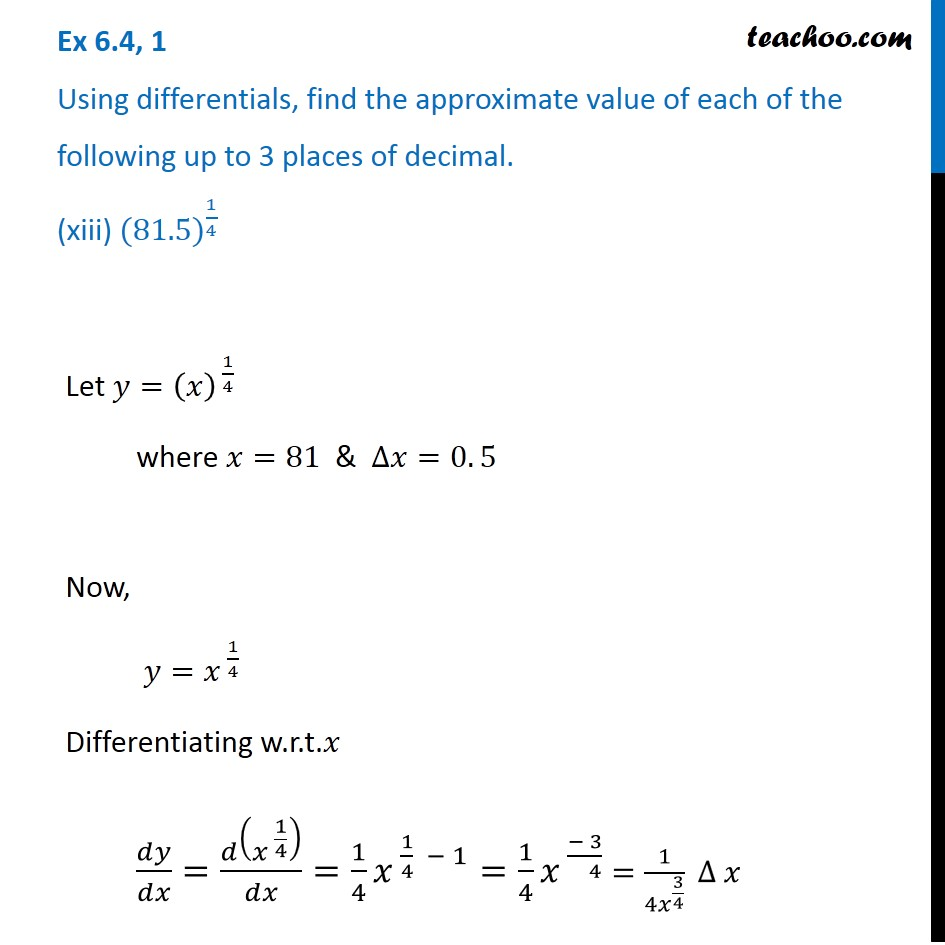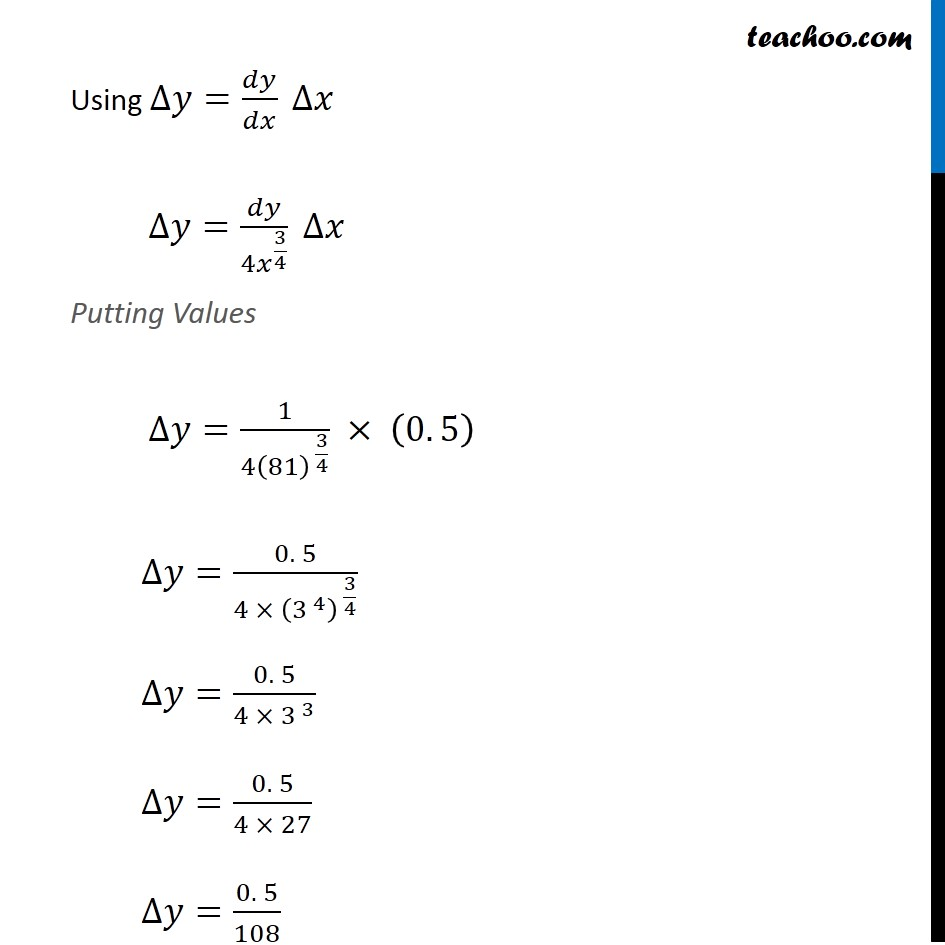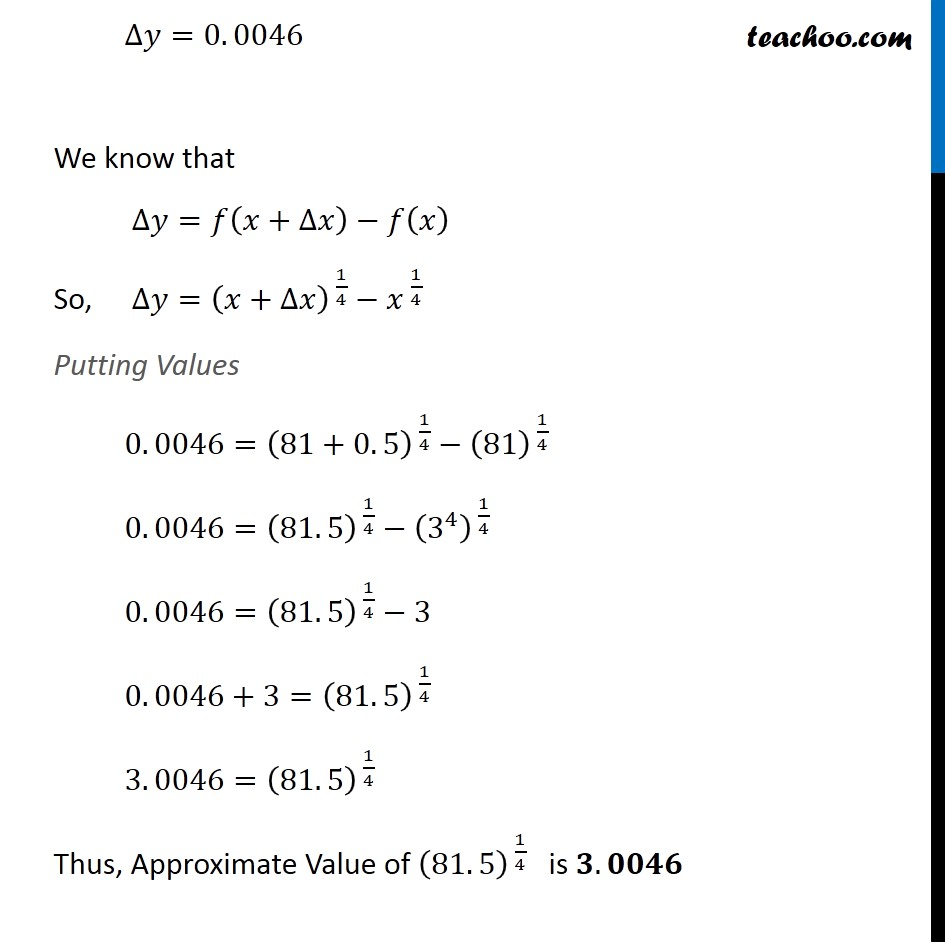Check Full Chapter Explained - Continuity and Differentiability - Application of Derivatives (AOD) Class 121. Chapter 6 Class 12 Application of Derivatives
2. Serial order wise
3. Ex 6.4

Transcript

Ex 6.4, 1 Using differentials, find the approximate value of each of the following up to 3 places of decimal. (xiii) 〖(81.5)〗^(1/4) Let 𝑦=(𝑥)^( 1/4) where 𝑥=81 & ∆𝑥=0. 5 Now, 𝑦=𝑥^( 1/4) Differentiating w.r.t.𝑥 𝑑𝑦/𝑑𝑥=𝑑(𝑥^( 1/4) )/𝑑𝑥=1/4 𝑥^( 1/4 − 1)=1/4 𝑥^( (− 3)/( 4)) =1/(4𝑥^(3/4) ) ∆ 𝑥 Using ∆𝑦=𝑑𝑦/𝑑𝑥 ∆𝑥 ∆𝑦=𝑑𝑦/(4𝑥^(3/4) ) ∆𝑥 Putting Values ∆𝑦=1/(4(81)^( 3/4) ) × (0. 5) ∆𝑦=(0. 5)/(4 × (3^( 4) )^( 3/4) ) ∆𝑦=(0. 5)/(4 × 3^( 3) ) ∆𝑦=(0. 5)/(4 × 27) ∆𝑦=(0. 5)/108 ∆𝑦=0. 0046 We know that ∆𝑦=𝑓(𝑥+∆𝑥)−𝑓(𝑥) So, ∆𝑦=(𝑥+∆𝑥)^( 1/4)−𝑥^( 1/4) Putting Values 0. 0046=(81+0. 5)^( 1/4)−(81)^( 1/4) 0. 0046=(81. 5)^( 1/4)−(3^4 )^( 1/4) 0. 0046=(81. 5)^( 1/4)−3 0. 0046+3=(81. 5)^( 1/4) 3. 0046=(81. 5)^( 1/4) Thus, Approximate Value of (81. 5)^( 1/4) is 𝟑. 𝟎𝟎𝟒𝟔

Ex 6.4